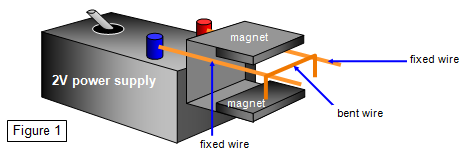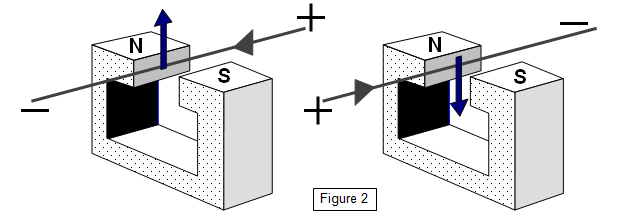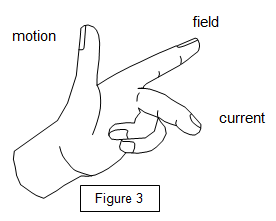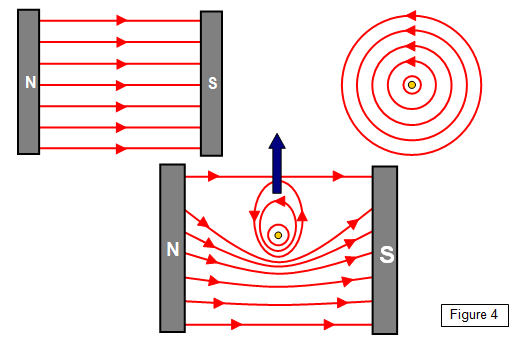# Motion from electricity

In 1821 Michael Faraday found a way of producing motion from electricity. He found that if a wire carrying an electric current was placed in a magnetic field then there was a force on the wire; and if it could it moved. This happens because of the combined effects of the magnetic fields of the wire and the magnets.

This may not seem very important but this simple fact is the basis of all our electric motors. Just think of how many times you use an electric motor in the house or at school – none of them would have been possible without Faraday's discovery.

The effect can easily be demonstrated by the experiment shown below (Figure 1).When a current is passed through the bent wire it moves along the two fixed wires between the poles of the magnets.
(Note : the magnets must be fixed to the metal yoke so that opposite poles face each other)

You will find that altering either the direction of the current or the direction of the magnetic field will change the direction of motion.

Figure 2 shows the arrangement clearly.The maximum force occurs when the current and the field are at right-angles to each other and the motion is then at right angles to both the field and the current.

The bigger the current and the stronger the magnetic field the greater the force on the wire and the faster it will move. Also the wire will 'feel' the biggest force if it is at right angles to the magnetic field and no force at all if it is parallel to the field.

Professor J .A. Fleming found a simple way of remembering the direction of motion using your left hand. It is called Fleming's Left Hand Rule. If the thumb, first finger and second finger of the left hand are placed at right angles to each other then:The First finger gives the Field (N to S)

The seCond finger gives the Current (+ to -)

The thuMb gives the Motion

## The catapult field

We will now look at the magnetic field that gives this motion; because of its shape it is called the CATAPULT FIELD.

The diagrams show the field of two flat magnets (i), the field of a wire (ii), and the result of putting the wire and magnets together (iii). It is this third field that is called the catapult field. You can see that the magnetic lines of force are close together near the wire so forcing it upwards. It is rather like the effect of a stretched rubber sheet on an object put on it.

(Think about the forces on a trampolinist when they are standing on a trampoline).

If the current direction is reversed the wire will be forced downwards.## schoolphysics catapult field animation

To see an animation of the catapult field click on the animation link.

## Force on a coil of wire in a magnetic fieldIf a coil of wire carrying a current is in a magnetic field then the way the current flows along one side of the coil will be opposite to the way it flows along the other side. (See Figure 5).

This means that one side of the coil will be pushed upwards and the other side pushed downwards and so the coil will twist. This rotation of the coil is the basis of all electric meters and motors.

With more turns of wire on the coil the twisting force will be greater.

A VERSION IN WORD IS AVAILABLE ON THE SCHOOLPHYSICS USB